STAT 330: 95-3

Final Examination: 9 December 1995 Partial Solutions

Instructor: Richard Lockhart

INSTRUCTIONS: This is an open book exam. You may use notes, text and other books as well as a calculator. You MUST be clear about what you are doing; if not I will assume you don't know what you are doing and mark accordingly. The exam is out of 77.

1. The attached SAS output, A, shows parts of the input and output for the analysis of the results of the following experiment. A total of 48 animals were each given one of 3 poisons, labelled I, II and III, so that 16 animals were given each poison. Each animal was then treated with 1 of 4 treatments, labelled A, B, C and D. Animals were assigned at random to one of the 12 possible poison/treatment combinations. For each animal, the response measured was time to death. The output analyzes the rate of dying defined to be 1 over the time to death.

1. From the incomplete output produce a complete ANOVA table for the analysis of this data set. [5 marks]

Solution: You fill in the ANOVA table for a two way layout with replications.

                            Sum of    MS     F
Source              DF     Squares

POISON               2      34.88   17.44  72.77
TREATMNT             3      20.41    6.80  28.33
POISON*TREATMNT      6       1.57    0.26   1.08
Error               36       8.65    0.24
Corrected Total     47      65.51
You cannot produce P values.
2. State and test relevant hypotheses, describing conclusions in real world terms in so far as possible. You will have to do fixed level testing in this problem. [5 marks]

Solution: The hypothesis of no interaction between the poison and the treatment is accepted. Those of no main effect of poison and no main effect for treatment are rejected even at the 1% level.

3. Which treatment is most effective at reducing the death rate? [2 marks]

Solution: Tukey's intervals show that treatment's B and D give definitely lower rates than A or C. The comparison of D to B is not significant but the estimated rate is lowest for B.

4. In SAS output B you will see the full ANOVA table for the original response variable, time to death. Why did I transform to do the analysis? [1 marks]

Solution: The answer, which was hard for students to come up with, is that there is less evidence of interactions in the transformed data.

5. What is the fitted residual corresponding to the first response in the cell Poison=I and Treatment=A (i.e. the value 0.31 at the top of the data table) for the model fitted in (d) above. [2 marks]

Solution: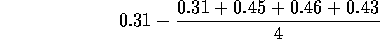2. Consider the following experiment. From each of 11 litters two rats are selected. One of the two is picked at random and raised in isolation while the other is raised in a cage with other rats and enriched'' surroundings. The rats are sacrificed on reaching adulthood and the cortexes are weighed. The data are analyzed two ways on SAS outputs C and D.

1. Does isolation reduce cortex weight? [5 marks]

Solution: This is paired comparisons; output D gives a two sided P value of 0.0088; the test is one sided so P=0.0044. In any case there is clear evidence of a reduction in cortex weight.

2. About how much effect on cortex weight does isolation have? Give an approximate 80% confidence interval. [5 marks]

Solution: from output D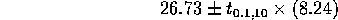3. A popular model in fisheries predicts that, except for error, the number y of fish returning to spawn is proportional to some power of x the number of young salmon (smolts) escaping'' 4 years previously. In symbols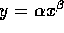. After taking logarithms this can be written as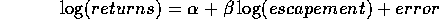This model is fitted to the data shown in SAS output E.

1. Give a 95% confidence interval for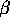[5 marks]

Solution: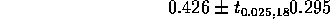2. Isless than 1? (This is an important scientific question.) [5 marks]

Solution: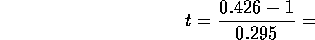Get P values from t tables with 18 degrees of freedom.

4. Four variations, labelled A, B, C, and D of a process for making penicillin are investigated. One of the basic ingredients is corn liquor. This liquor must be blended in batches. The batches are big enough to split into 4 and so the experiment is run as follows. Five batches of the liquor are made. Each is split into 4 parts and each of the four parts is used with one of the 4 variations of the process. Each variation is tried on exactly one of the 4 parts of each batch of liquor. The yield of penicillin is then recorded for each of the resulting 20 runs. The data and output of 2 SAS models (both done in one run) are in SAS output F.

1. Is there a difference between the four variations of the process in terms of average yield? [4 marks]

Solution: This is a randomized complete blocks design. The first model fitted is a one way layout model which is wrong. The two way additive model shows an F statistic of 3.96 for treatment; the corresponding P value is 0.0229. There is relatively good evidence for a difference.

2. Which variant produces the highest yield? [2 marks]

Solution: Use the Tukey intervals! The intervals show that while treatment C had the highest mean you cannot be sure that C is the highest yield.

5. A new process for measuring the concentration of a chemical in water is being investigated. A total of n samples are prepared in which the concentrations are the known numbers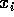for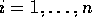; the new process is used to measure the concentrations for these samples. It is thought likely that the concentrations measured by the new process, which we denote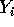, will be related to the true concentrations via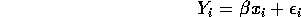where the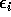are independent, have mean 0 and all have the same variance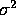which is unknown.

1. If this model is fitted by least squares, (that is by minimizing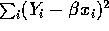) show that the least squares estimate ofis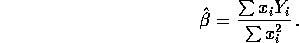[5 marks]

Solution: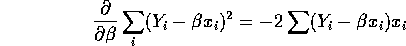Set this equal to 0 to get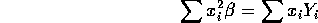Solve forto get the desired answer.

2. Show that the estimator in part (a) is unbiased. [4 marks]

Solution: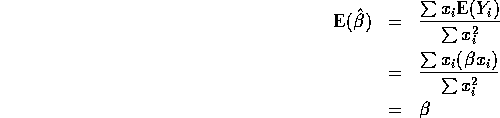3. Compute (give a formula for) the standard error of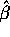. [4 marks]

Solution: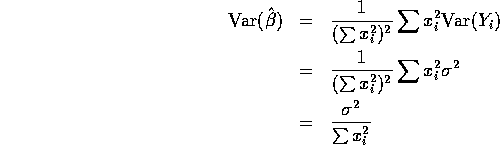So the standard error ofis the square root of the variance or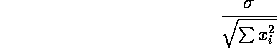4. The error sum of squares for this model is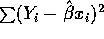which may be shown to have n-1 degrees of freedom. If theare the numbers 1, 2, 3 and 4,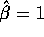and the error sum of squares is 0.12 find a 95% confidence interval forand explain what further assumptions you must make to do so. [4 marks]

Solution: The estimate of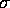is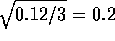. The estimated standard error ofis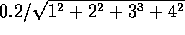which is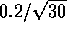so the confidence interval is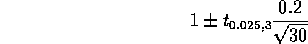6. A simple random sample is drawn from a large population and p is the proportion of that population favouring sovereignty for Québec.

1. If the true value of p is 0.496 then how big does the sample need to be to give an 80% chance of rejecting the hypothesis p>=0.5 at the level 0.05. [5 marks]

Solution: Use the formula for N on the bottom of page 329 for a one-tailed test with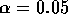,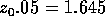,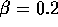,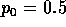and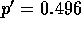.

2. If the true value of p is 0.496 what is the probability that a survey of 1600 people will have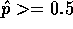? [5 marks]

NOTE: You may assume the population is very large so that there is no harm in pretending the sampling is carried out with replacement.

Solution: X is Binomial(1600,0.496) and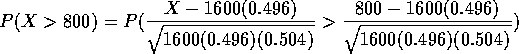which is the area to the right of 0.32 under a standard normal curve or 0.374 approximately.

7. A sample of 50 people is divided into 5 groups of 10 people each. Four of the groups are put on special diets; the fifth serves as control. After 13 weeks the blood concentration of high density lipoprotein (HDL) is measured for each person. Data (hypothetical), sas commands and output are in SAS output G.

1. If the average concentration of HDL in the population at large is reported in the literature to be 1.24 could sampling fluctuation for simple random sampling explain the difference between the control average and this reported value? [4 marks]

Solution: You are supposed to test the hypothesis that the control mean is 1.24 based on a sample of size 10 with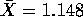and s=0.116. This leads to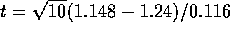with 9 degrees of freedom.

2. Are there any differences between the diets (including control)? [4 marks]

Solution: This is a 1 way layout problem. The P value for the F test is 0.0077 which is quite decidedly significant so, yes, there are differences.

3. Which diets lead to the lowest level of HDL? [2 marks]

Solution: The Tukey intervals show that Diets A and B are clearly lower than the control level. They do not distinguish clearly between the other 4 diets.

DATA

0.31 I A
0.45 I A
0.46 I A
0.43 I A
0.82 I B
1.10 I B
0.88 I B
0.72 I B
0.43 I C
0.45 I C
0.63 I C
0.76 I C
0.45 I D
0.71 I D
0.66 I D
0.62 I D
0.36 II A
0.29 II A
0.40 II A
0.23 II A
0.92 II B
0.61 II B
0.49 II B
1.24 II B
0.44 II C
0.35 II C
0.31 II C
0.40 II C
0.56 II D
1.02 II D
0.71 II D
0.38 II D
0.22 III A
0.21 III A
0.18 III A
0.23 III A
0.30 III B
0.37 III B
0.38 III B
0.29 III B
0.23 III C
0.25 III C
0.24 III C
0.22 III C
0.30 III D
0.36 III D
0.31 III D
0.33 III D
CODE
  options pagesize=60 linesize=80;
data poison;
infile 'poison.dat';
input time poison $treatmnt$ ;
rate=1/time;
proc glm data=poison;
class poison treatmnt;
model rate = poison|treatmnt;
means treatmnt / tukey cldiff alpha=0.05;
means treatmnt / tukey ;
run;
OUTPUT
                        General Linear Models Procedure
Dependent Variable: RATE
Sum of
Source                  DF     Squares

POISON                          34.88
TREATMNT                        20.41
POISON*TREATMNT                  1.57

Corrected Total         47      65.51

R-Square             C.V.        Root MSE            RATE Mean
0.868055         18.68478       0.4899853            2.6223763

Tukey's Studentized Range (HSD) Test for variable: RATE
Alpha= 0.05  Confidence= 0.95  df= 36  MSE= 0.240086
Critical Value of Studentized Range= 3.809
Minimum Significant Difference= 0.5387

Comparisons significant at the 0.05 level are indicated by '***'.

Simultaneous            Simultaneous
Lower    Difference     Upper
TREATMNT      Confidence    Between   Confidence
Comparison        Limit       Means       Limit

A    - C          0.0334      0.5721      1.1109   ***
A    - D          0.8196      1.3583      1.8971   ***
A    - B          1.1187      1.6574      2.1961   ***

C    - A         -1.1109     -0.5721     -0.0334   ***
C    - D          0.2475      0.7862      1.3249   ***
C    - B          0.5465      1.0853      1.6240   ***

D    - A         -1.8971     -1.3583     -0.8196   ***
D    - C         -1.3249     -0.7862     -0.2475   ***
D    - B         -0.2397      0.2991      0.8378

B    - A         -2.1961     -1.6574     -1.1187   ***
B    - C         -1.6240     -1.0853     -0.5465   ***
B    - D         -0.8378     -0.2991      0.2397

Tukey's Studentized Range (HSD) Test for variable: RATE

Alpha= 0.05  df= 36  MSE= 0.240086
Critical Value of Studentized Range= 3.809
Minimum Significant Difference= 0.5387

Means with the same letter are not significantly different.

Tukey Grouping              Mean      N  TREATMNT

A            3.5193     12  A

B            2.9472     12  C

C            2.1610     12  D
C
C            1.8619     12  B

CODE

  options pagesize=60 linesize=80;
data poison;
infile 'poison.dat';
input time poison $treatmnt$ ;
proc glm data=poison;
class poison treatmnt;
model time = poison|treatmnt;
means treatmnt / tukey cldiff alpha=0.05;
means treatmnt / tukey ;
run;
OUTPUT
                        General Linear Models Procedure
Dependent Variable: TIME
Sum of            Mean
Source                  DF          Squares          Square   F Value     Pr > F

Model                   11       2.20435625      0.20039602      9.01     0.0001
Error                   36       0.80072500      0.02224236
Corrected Total         47       3.00508125

R-Square             C.V.        Root MSE            TIME Mean
0.733543         31.11108       0.1491387            0.4793750

Source                  DF        SS            Mean Square   F Value     Pr > F

POISON                   2       1.03301250      0.51650625     23.22     0.0001
TREATMNT                 3       0.92120625      0.30706875     13.81     0.0001
POISON*TREATMNT          6       0.25013750      0.04168958      1.87     0.1123

Tukey's Studentized Range (HSD) Test for variable: TIME

Alpha= 0.05  Confidence= 0.95  df= 36  MSE= 0.022242
Critical Value of Studentized Range= 3.809
Minimum Significant Difference= 0.164

Simultaneous            Simultaneous
Lower    Difference     Upper
TREATMNT      Confidence    Between   Confidence
Comparison        Limit       Means       Limit

B    - D        -0.02148     0.14250     0.30648
B    - C         0.12019     0.28417     0.44815   ***
B    - A         0.19852     0.36250     0.52648   ***

D    - B        -0.30648    -0.14250     0.02148
D    - C        -0.02231     0.14167     0.30565
D    - A         0.05602     0.22000     0.38398   ***

C    - B        -0.44815    -0.28417    -0.12019   ***
C    - D        -0.30565    -0.14167     0.02231
C    - A        -0.08565     0.07833     0.24231

A    - B        -0.52648    -0.36250    -0.19852   ***
A    - D        -0.38398    -0.22000    -0.05602   ***
A    - C        -0.24231    -0.07833     0.08565

Tukey's Studentized Range (HSD) Test for variable: TIME

Alpha= 0.05  df= 36  MSE= 0.022242
Critical Value of Studentized Range= 3.809
Minimum Significant Difference= 0.164

Means with the same letter are not significantly different.

Tukey Grouping              Mean      N  TREATMNT

A           0.67667     12  B
A
B       A           0.53417     12  D
B
B       C           0.39250     12  C
C
C           0.31417     12  A

DATA

Enriched 689
Isolated 657
Enriched 656
Isolated 623
Enriched 668
Isolated 652
Enriched 660
Isolated 654
Enriched 679
Isolated 658
Enriched 663
Isolated 646
Enriched 664
Isolated 600
Enriched 647
Isolated 640
Enriched 694
Isolated 605
Enriched 633
Isolated 635
Enriched 653
Isolated 642

The SAS code

  options pagesize=60 linesize=80;
data cortex;
infile 'cortex.dat';
input treatmnt $weight ; proc sort data=cortex; by treatmnt; proc ttest cochran; class treatmnt; run; The output  TTEST PROCEDURE Variable: WEIGHT TREATMNT N Mean Std Dev Std Error ------------------------------------------------------------------------------- Enriched 11 664.18181818 17.93777122 5.40844152 Isolated 11 637.45454545 20.15124630 6.07582937 Variances T Method DF Prob>|T| -------------------------------------------------------- Unequal 3.2857 Satterthwaite 19.7 0.0037 Cochran 10.0 0.0082 Equal 3.2857 20.0 0.0037 For H0: Variances are equal, F' = 1.26 DF = (10,10) Prob>F' = 0.7200 DATA 689 657 656 623 668 652 660 654 679 658 663 646 664 600 647 640 694 605 633 635 653 642 The SAS code  options pagesize=60 linesize=80; data cortexpr; infile 'cortexpr.dat'; input enriched isolated; diff=enriched-isolated; proc means mean std stderr t prt maxdec=2; run; The output  Variable Mean Std Dev Std Error T Prob>|T| -------------------------------------------------------------------------- ENRICHED 664.18 17.94 5.41 122.80 0.0001 ISOLATED 637.45 20.15 6.08 104.92 0.0001 DIFF 26.73 27.33 8.24 3.24 0.0088 -------------------------------------------------------------------------- The data follow. The fourth column is the logarithm of the second and the fifth is the log of the third. The second column is the number of returning adults in millions and the third is the number of smolts escaping in millions. 1960 1.154 14.4 0.1432342 2.667228 1961 1.159 25.0 0.1475576 3.218876 1962 1.422 24.6 0.3520643 3.202746 1963 2.156 32.7 0.7682547 3.487375 1964 2.455 28.7 0.8981268 3.356897 1965 2.576 31.9 0.9462378 3.462606 1966 3.160 30.2 1.1505720 3.407842 1967 2.429 35.2 0.8874797 3.561046 1968 1.818 31.6 0.5977370 3.453157 1969 3.005 41.5 1.1002776 3.725693 1970 3.974 33.6 1.3797731 3.514526 1971 2.162 40.7 0.7710337 3.706228 1972 2.164 47.7 0.7719584 3.864931 1973 3.256 42.0 1.1804994 3.737670 1974 1.916 39.6 0.6502397 3.678829 1975 4.272 41.8 1.4520821 3.732896 1976 1.190 42.7 0.1739533 3.754199 1977 1.859 39.7 0.6200387 3.681351 1978 1.778 53.1 0.5754891 3.972177 1979 1.422 46.9 0.3520643 3.848018 The SAS code  data fish; infile 'fish.dat'; input year adults smolts logretrn logescap ; proc reg; model logretrn = logescap; run; The output Model: MODEL1 Dependent Variable: LOGRETRN Analysis of Variance Sum of Mean Source DF Squares Square F Value Prob>F Model 1 0.30002 0.30002 2.078 0.1666 Error 18 2.59839 0.14436 C Total 19 2.89841 Root MSE 0.37994 R-square 0.1035 Dep Mean 0.74593 Adj R-sq 0.0537 C.V. 50.93494 Parameter Estimates Parameter Standard T for H0: Variable DF Estimate Error Parameter=0 Prob > |T| INTERCEP 1 -0.766287 1.05238687 -0.728 0.4759 LOGESCAP 1 0.425772 0.29533681 1.442 0.1666 DATA 1 A 86 1 B 86 1 C 97 1 D 96 2 A 81 2 B 75 2 C 92 2 D 81 3 A 78 3 B 85 3 C 87 3 D 87 4 A 84 4 B 90 4 C 89 4 D 86 5 A 76 5 B 79 5 C 80 5 D 90 The code  options pagesize=60 linesize=80; data pen; infile 'pen.dat'; input blend treatmnt$ yield;
proc glm data=pen;
class blend treatmnt ;
model yield = treatmnt;
means treatmnt / tukey cldiff alpha=0.05;
means treatmnt / tukey ;
proc glm data=pen;
class blend treatmnt ;
model yield = treatmnt blend;
means treatmnt / tukey cldiff alpha=0.05;
means treatmnt / tukey ;
run;
end{verbatim}
The output
\begin{verbatim}
General Linear Models Procedure
Dependent Variable: YIELD
Sum of            Mean
Source                  DF          Squares          Square   F Value     Pr > F

Model                    3     223.75000000     74.58333333      2.44     0.1025
Error                   16     490.00000000     30.62500000

Corrected Total         19     713.75000000
R-Square             C.V.        Root MSE           YIELD Mean
0.313485         6.491479       5.5339859            85.250000

Source                  DF        Type I SS     Mean Square   F Value     Pr > F

TREATMNT                 3     223.75000000     74.58333333      2.44     0.1025

Tukey's Studentized Range (HSD) Test for variable: YIELD

NOTE: This test controls the type I experimentwise error rate.

Alpha= 0.05  Confidence= 0.95  df= 16  MSE= 30.625
Critical Value of Studentized Range= 4.046
Minimum Significant Difference= 10.014

Comparisons significant at the 0.05 level are indicated by '***'.

Simultaneous            Simultaneous
Lower    Difference     Upper
TREATMNT      Confidence    Between   Confidence
Comparison        Limit       Means       Limit

C    - D          -9.014       1.000      11.014
C    - B          -4.014       6.000      16.014
C    - A          -2.014       8.000      18.014

D    - C         -11.014      -1.000       9.014
D    - B          -5.014       5.000      15.014
D    - A          -3.014       7.000      17.014

B    - C         -16.014      -6.000       4.014
B    - D         -15.014      -5.000       5.014
B    - A          -8.014       2.000      12.014

A    - C         -18.014      -8.000       2.014
A    - D         -17.014      -7.000       3.014
A    - B         -12.014      -2.000       8.014

General Linear Models Procedure

Tukey's Studentized Range (HSD) Test for variable: YIELD

NOTE: This test controls the type I experimentwise error rate, but
generally has a higher type II error rate than REGWQ.

Alpha= 0.05  df= 16  MSE= 30.625
Critical Value of Studentized Range= 4.046
Minimum Significant Difference= 10.014

Means with the same letter are not significantly different.

Tukey Grouping              Mean      N  TREATMNT

A            89.000      5  C
A
A            88.000      5  D
A
A            83.000      5  B
A
A            81.000      5  A

General Linear Models Procedure
Dependent Variable: YIELD
Sum of            Mean
Source                  DF          Squares          Square   F Value     Pr > F

Model                    7     487.75000000     69.67857143      3.70     0.0229
Error                   12     226.00000000     18.83333333

Corrected Total         19     713.75000000
R-Square             C.V.        Root MSE           YIELD Mean
0.683363         5.090603       4.3397389            85.250000

Source                  DF        Type I SS     Mean Square   F Value     Pr > F

TREATMNT                 3     223.75000000     74.58333333      3.96     0.0356
BLEND                    4     264.00000000     66.00000000      3.50     0.0407

Tukey's Studentized Range (HSD) Test for variable: YIELD

NOTE: This test controls the type I experimentwise error rate.

Alpha= 0.05  Confidence= 0.95  df= 12  MSE= 18.83333
Critical Value of Studentized Range= 4.199
Minimum Significant Difference= 8.1485

Comparisons significant at the 0.05 level are indicated by '***'.

Simultaneous            Simultaneous
Lower    Difference     Upper
TREATMNT      Confidence    Between   Confidence
Comparison        Limit       Means       Limit

C    - D          -7.149       1.000       9.149
C    - B          -2.149       6.000      14.149
C    - A          -0.149       8.000      16.149

D    - C          -9.149      -1.000       7.149
D    - B          -3.149       5.000      13.149
D    - A          -1.149       7.000      15.149

B    - C         -14.149      -6.000       2.149
B    - D         -13.149      -5.000       3.149
B    - A          -6.149       2.000      10.149

A    - C         -16.149      -8.000       0.149
A    - D         -15.149      -7.000       1.149
A    - B         -10.149      -2.000       6.149

General Linear Models Procedure

Tukey's Studentized Range (HSD) Test for variable: YIELD

NOTE: This test controls the type I experimentwise error rate, but
generally has a higher type II error rate than REGWQ.

Alpha= 0.05  df= 12  MSE= 18.83333
Critical Value of Studentized Range= 4.199
Minimum Significant Difference= 8.1485

Means with the same letter are not significantly different.

Tukey Grouping              Mean      N  TREATMNT

A            89.000      5  C
A
A            88.000      5  D
A
A            83.000      5  B
A
A            81.000      5  A

DATA

 1.064  Control
1.221 Control
1.053 Control
1.123  Control
0.989 Control
1.142 Control
1.110 Control
1.247 Control
1.132 Control
1.399 Control
0.997 Diet_A
0.998 Diet_A
0.920 Diet_A
1.240 Diet_A
0.778 Diet_A
0.970 Diet_A
0.909 Diet_A
1.046 Diet_A
0.865 Diet_A
0.845 Diet_A
0.810 Diet_B
0.897 Diet_B
1.088 Diet_B
1.006 Diet_B
1.121 Diet_B
1.054 Diet_B
0.822 Diet_B
1.039 Diet_B
0.756 Diet_B
1.125 Diet_B
0.983 Diet_C
1.041 Diet_C
0.834 Diet_C
0.947 Diet_C
1.105 Diet_C
1.218  Diet_C
0.980 Diet_C
1.003 Diet_C
0.984 Diet_C
1.104 Diet_C
0.993 Diet_D
0.855 Diet_D
1.004  Diet_D
1.069 Diet_D
0.941 Diet_D
1.103 Diet_D
0.941 Diet_D
1.001 Diet_D
1.240 Diet_D
1.074 Diet_D
  options pagesize=60 linesize=80;
data hdl;
infile 'hdl.dat';
input hdl diet \$  ;
proc means;
by diet;
proc glm data=hdl;
class diet ;
model hdl = diet;
means diet / tukey cldiff alpha=0.05;
run;
           Analysis Variable : HDL
---------------------------------- DIET=Control --------------------------------

N          Mean       Std Dev       Minimum       Maximum
----------------------------------------------------------
10     1.1480000     0.1163586     0.9890000     1.3990000
----------------------------------------------------------

---------------------------------- DIET=Diet_A ---------------------------------

N          Mean       Std Dev       Minimum       Maximum
----------------------------------------------------------
10     0.9568000     0.1283145     0.7780000     1.2400000
----------------------------------------------------------

---------------------------------- DIET=Diet_B ---------------------------------

N          Mean       Std Dev       Minimum       Maximum
----------------------------------------------------------
10     0.9718000     0.1384275     0.7560000     1.1250000
----------------------------------------------------------

---------------------------------- DIET=Diet_C ---------------------------------

N          Mean       Std Dev       Minimum       Maximum
----------------------------------------------------------
10     1.0199000     0.1045652     0.8340000     1.2180000
----------------------------------------------------------

---------------------------------- DIET=Diet_D ---------------------------------

N          Mean       Std Dev       Minimum       Maximum
----------------------------------------------------------
10     1.0221000     0.1062570     0.8550000     1.2400000
----------------------------------------------------------

General Linear Models Procedure
Dependent Variable: HDL
Sum of            Mean
Source                  DF          Squares          Square   F Value     Pr > F

Model                    4       0.22636708      0.05659177      3.96     0.0077
Error                   45       0.64251500      0.01427811

Corrected Total         49       0.86888208
R-Square             C.V.        Root MSE             HDL Mean
0.260527         11.67224       0.1194911            1.0237200

Source                  DF     Sum of Squares   Mean Square   F Value     Pr > F
DIET                     4       0.22636708      0.05659177      3.96     0.0077

General Linear Models Procedure
Tukey's Studentized Range (HSD) Test for variable: HDL
NOTE: This test controls the type I experimentwise error rate.

Alpha= 0.05  Confidence= 0.95  df= 45  MSE= 0.014278
Critical Value of Studentized Range= 4.018
Minimum Significant Difference= 0.1518

Comparisons significant at the 0.05 level are indicated by '***'.

Simultaneous            Simultaneous
Lower    Difference     Upper
DIET           Confidence    Between   Confidence
Comparison           Limit       Means       Limit

Control - Diet_D      -0.02594     0.12590     0.27774
Control - Diet_C      -0.02374     0.12810     0.27994
Control - Diet_B       0.02436     0.17620     0.32804   ***
Control - Diet_A       0.03936     0.19120     0.34304   ***

Diet_D  - Control     -0.27774    -0.12590     0.02594
Diet_D  - Diet_C      -0.14964     0.00220     0.15404
Diet_D  - Diet_B      -0.10154     0.05030     0.20214
Diet_D  - Diet_A      -0.08654     0.06530     0.21714

Diet_C  - Control     -0.27994    -0.12810     0.02374
Diet_C  - Diet_D      -0.15404    -0.00220     0.14964
Diet_C  - Diet_B      -0.10374     0.04810     0.19994
Diet_C  - Diet_A      -0.08874     0.06310     0.21494

Diet_B  - Control     -0.32804    -0.17620    -0.02436   ***
Diet_B  - Diet_D      -0.20214    -0.05030     0.10154
Diet_B  - Diet_C      -0.19994    -0.04810     0.10374
Diet_B  - Diet_A      -0.13684     0.01500     0.16684

Diet_A  - Control     -0.34304    -0.19120    -0.03936   ***
Diet_A  - Diet_D      -0.21714    -0.06530     0.08654
Diet_A  - Diet_C      -0.21494    -0.06310     0.08874
Diet_A  - Diet_B      -0.16684    -0.01500     0.13684